Courses

Test: 35 Year JEE Previous Year Questions: Work, Energy and Power

26 Questions MCQ Test Physics 35 Years JEE Main & Advanced Past year Papers | Test: 35 Year JEE Previous Year Questions: Work, Energy and Power

Description
Attempt Test: 35 Year JEE Previous Year Questions: Work, Energy and Power | 26 questions in 50 minutes | Mock test for JEE preparation | Free important questions MCQ to study Physics 35 Years JEE Main & Advanced Past year Papers for JEE Exam | Download free PDF with solutions
QUESTION: 1

Consider the following two statements : A Linear momentum of a system of particles is zero B. Kinetic energy of a system of particles is zero.Then

Solution:

Kinetic energy of a system of particle is zero only when the speed of each particles is zero. And if speed of each particle is zero, the linear momentum of the system of particle has to be zero.
Also the linear momentum of the system may be zero even when the particles are moving. This is because linear momentum is a vector quantity. In this case the kinetic energy of the system of particles will not be zero.

∴ A does not imply B but B implies A.

QUESTION: 2

A wire suspended vertically from one of  its ends is stretched by attaching a weight of 200N to the lower end.  The weight stretches the wire by 1 mm. Then the elastic energy stored in the wire is

Solution:

The elastic potential energy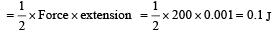QUESTION: 3

A spring of spring constant 5 × 103 N/m is stretched initially by 5cm from the unstretched position. Then the work required to stretch it further by another 5 cm is

Solution:

k = 5*103 N/m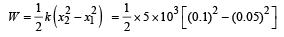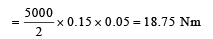QUESTION: 4

A body is moved  along a straight line by a machine delivering a constant power.  The distance moved by the body in time ‘t’ is proportional to

Solution:

We know that  F × v = Power

∴ F ´ v=c   where c = constant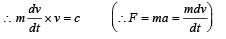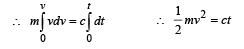.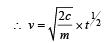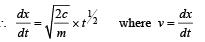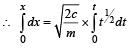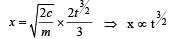QUESTION: 5

A particle moves in a straight line with retar dation proportional to its displacement. Its loss of kinetic energy for any displacement x is proportional to

Solution:

Given : retardation µ displacement

i.e., a =-kx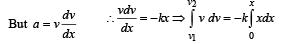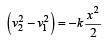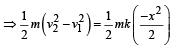∴ Loss in kinetic energy,∴ DKαx 2

QUESTION: 6

A uniform chain of length 2 m is kept on a table such that a length of 60 cm hangs freely from the edge of the table. The total mass of the chain is 4 kg. What is the work done in pulling the entire chain on the table ?

Solution:

Mass of over hanging chain  m‘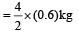Let at the surface PE = 0

C.M.of hanging part = 0.3 m below the table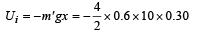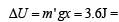Workdone in putting the en tire chain on the table.

QUESTION: 7

A force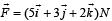is applied over a particle which displaces it from its origin to the point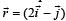m. The work done on the particle in joules is

Solution:

Workdone in displacing the particle,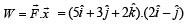= 10 – 3 = 7 joules

QUESTION: 8

A body of mass ‘m’, accelerates uniformly from rest to ‘v1’ in time ‘t1’. The instantaneous power delivered to the body as a function of time ‘t’ is

Solution:

Let acceleration of body be a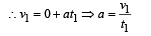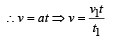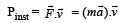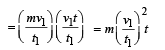QUESTION: 9

A Particle is acted upon by a force of constant magnitude which is always perpendicular to the velocity of the particle, the motion of the particles takes place in a plane. It follows that

Solution:

Work done by such force is always zero since force is acting in a direction perpendicular to velocity. ∴ from work-energy theorem = ΔK=0 K remains constant.

QUESTION: 10

The block of mass M moving on the frictionless horizontal surface collides with the spring of spring constant k and compresses it by length L. The maximum momentum of the block after collision is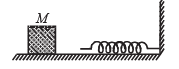Solution: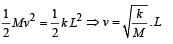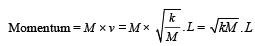QUESTION: 11

A spherical ball of mass 20 kg is stationary at the top of a hill of height 100 m. It rolls down a smooth surface to the ground, then climbs up another hill of height 30 m and finally rolls down to a horizontal base at a height of 20 m above the ground. The velocity attained by the ball is

Solution: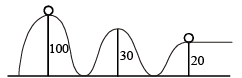Loss in potential energy = gain in kinetic energy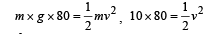v2 = 1600   or   v = 40 m/s

QUESTION: 12

A body of mass m is accelerated uniformly from rest to a speed v in a time T. The instantaneous power delivered to the body as a function of time is given by

Solution:

u = 0; v = u + aT; v = aT

Instantaneous power = F × v = m. a. at = m.a2.t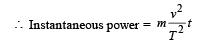QUESTION: 13

A particle of mass 100g is thrown vertically upwards with a speed of  5 m/s. The work done by the force of gravity during the time the particle goes up is

Solution: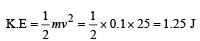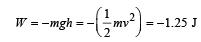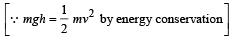QUESTION: 14

The potential energy of a 1 kg particle free to move along
the x-axis is given by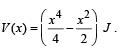The total mechanical energy of the particle is 2 J. Then, the maximum speed (in m/s) is

Solution:

Velocity is maximum when K.E. is maximum For minimum. P.E.,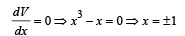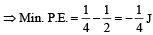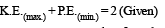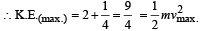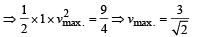QUESTION: 15

A 2 kg block slides on a horizontal floor with a speed of 4m/s.It strikes a uncompressed spring, and compresses it till the block is motionless. The kinetic friction force is 15N and spring constant is 10,000 N/m. The spring compresses by

Solution:

Let the block compress the spring by x before stopping. kinetic energy of the block = (P.E of compressed spring) + work done against friction.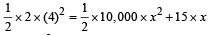10,000x2  + 30x – 32 = 0

⇒ 5000x2 + 15x - 16= 0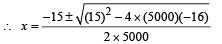= 0.055m = 5.5cm.

QUESTION: 16

An athlete in the olympic games covers a distance of 100 m in 10s. His kinetic energy can be estimated to be in the range

Solution:

The average speed of the athelete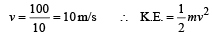If mass is 40 kg then,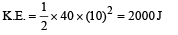If mass is 100 kg then, K.E.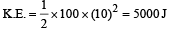QUESTION: 17

A block of mass 0.50 kg is moving with a speed of 2.00 ms–1 on a smooth surface. It strikes another mass of 1.00 kg and then they move together as a single body. The energy loss during the collision is

Solution:

Initial  kinetic energy of the system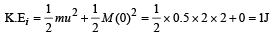For collision, applying conservation of linear momentum m × u = (m + M) × v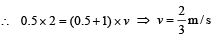Final kinetic energy of the system is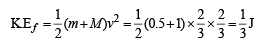∴ Energy loss during collision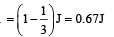QUESTION: 18

The potential energy function for the force between two atoms in a diatomic molecule is approximately given by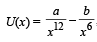where a and b are constants and x is the distance between the atoms. If the dissociation energy of the molecule is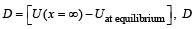is

Solution:

At equilibrium :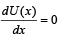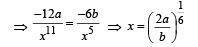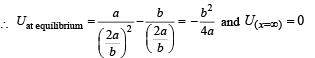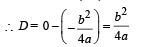QUESTION: 19

This question has Statement 1 and Statement 2. Of the four choices given after the Statements, choose the one that best describes the two Statements.
If two springs S1 and S2 of force constants k1 and k2, respectively, are stretched by the same force, it is found that more work is done on spring S1 than on spring S2.
STATEMENT 1 : If stretched by the same amount work done on S1, Work done on S1 is more than S

STATEMENT 2 : k1 < k2

Solution:

When force is same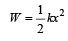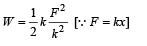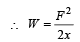.

As W1 > W2

∴ k1 < k2

When extension is same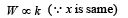∴ W1 < W2

Statement 1 is false and statement 2 is true.

QUESTION: 20

When a rubber-band is stretched by a distance x, it exerts restoring force of magnitude F = ax + bx2 where a and b are constants. The work done in stretching the unstretched rubber-band by L is:

Solution:

Given F = ax   +  bx2

According  to work-energy  theorem, we know that work done in stretching the rubber band by t is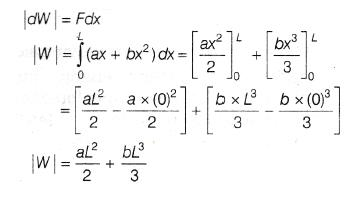QUESTION: 21

A person trying to lose weight by burning fat lifts a mass of 10 kg upto a height of 1 m 1000 times. Assume that the potential energy lost each time he lowers the mass is dissipated. How much fat will he use up considering the work done only when the weight is lifted up? Fat supplies 3.8 × 107 J of energy per kg which is converted to mechanical energy with a 20% efficiency rate. Take g = 9.8 ms–2 :

Solution: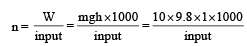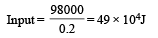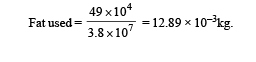QUESTION: 22

A small block of mass M moves on a frictionless surface of an inclined plane, as shown in figure. The angle of the incline suddenly changes from 60° to 30° at point B. The block is initially at rest at A. Assume that collisions between the block and the incline are totally inelastic (g = 10 m/s2).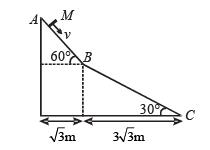Q.1. The speed of the block at point B immediately after it strikes the second incline is –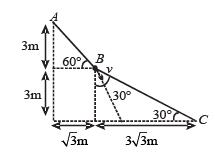Solution: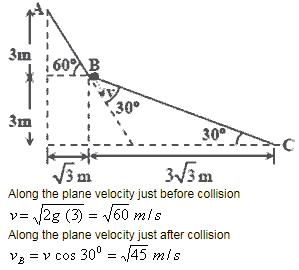QUESTION: 23

A small block of mass M moves on a frictionless surface of an inclined plane, as shown in figure. The angle of the incline suddenly changes from 60° to 30° at point B. The block is initially at rest at A. Assume that collisions between the block and the incline are totally inelastic (g = 10 m/s2).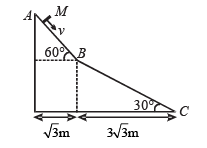Q.2. The speed of the block at point C, immediately before it leaves the second incline is

Solution: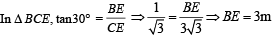Applying mechanical energy conservation .
Mechanical energy at B = Mechanical energy at C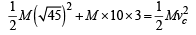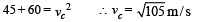QUESTION: 24

A small block of mass M moves on a frictionless surface of an inclined plane, as shown in figure. The angle of the incline suddenly changes from 60° to 30° at point B. The block is initially at rest at A. Assume that collisions between the block and the incline are totally inelastic (g = 10 m/s2).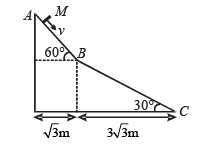Q.3. If collision between the block and the incline is completely elastic, then the vertical (upward) component of the velocity of the block at point B, immediately after it strikes the second incline is –

Solution:

The velocity of the block along BC just before collision is v cos 30°. The impact forces act perpendicular to the surface so the component of velocity along the incline remains unchanged.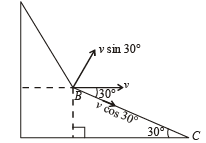Also since the collision is elastic, the vertical component of velocity (v sin 30°) before collision changes in direction, the magnitude remaining the same as shown in the figure. So the rectangular components of velocity after collision are as shown in the figure.
This means that the final velocity of the block should be horizontal making an angle 30º with BC. Therefore the vertical component of the final velocity of the block is zero.

QUESTION: 25

A small block of mass 1 kg is released from rest at the top of a rough track. The track is a circular arc of radius 40 m. The block slides along the track without toppling and a frictional force acts on it in the direction opposite to the instantaneous velocity. The work done in overcoming the friction up to the point Q, as shown in the figure below, is 150 J. (Take the acceleration due to gravity, g = 10 ms–2)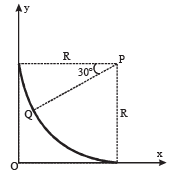Q.4. The magnitude of the normal reaction that acts on the block at the point Q is

Solution: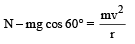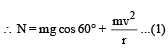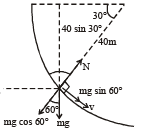Loss in P.E. = mg × 40 sin 30° = 200 J

Work done in over coming friction = 150 J

∴ K.E. possessed by the particle = 50 J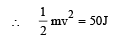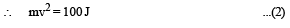From (1) and (2)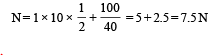(a) is the correct option.

QUESTION: 26

A small block of mass 1 kg is released from rest at the top of a rough track. The track is a circular arc of radius 40 m. The block slides along the track without toppling and a frictional force acts on it in the direction opposite to the instantaneous velocity. The work done in overcoming the friction up to the point Q, as shown in the figure below, is 150 J. (Take the acceleration due to gravity, g = 10 ms–2)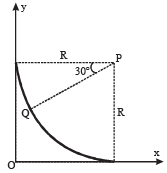Q.5.The speed of the block when it reaches the point Q is

Solution:

From (2), mv2 = 100  ∴ v = 10 ms–1

(b) is the correct option.Use Code STAYHOME200 and get INR 200 additional OFF Use Coupon Code

Track your progress, build streaks, highlight & save important lessons and more!

Similar ContentRelated tests